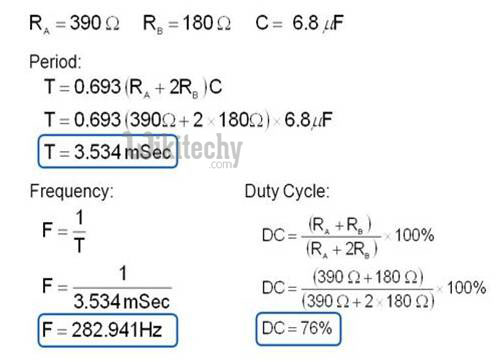# 555 Timer LED Flasher - Calculating the Pulse Rate

## Pulse Rate

• In astable mode, the output from the 555 timer is a continuous pulse waveform of a specific frequency that depends on the values of the two resistors (RA and RB) and capacitor (C) used in the circuit.Circuit Pulse

• The charge time (Output High)
• t1=0.693(R1+R2)C1
• And Output Low
• t2=0.693(R2)C1
• Total time period , T=t1+t2.
• Frequency , F=1/T.

## Duty Cycle CalculationDuty Cycle Calculation